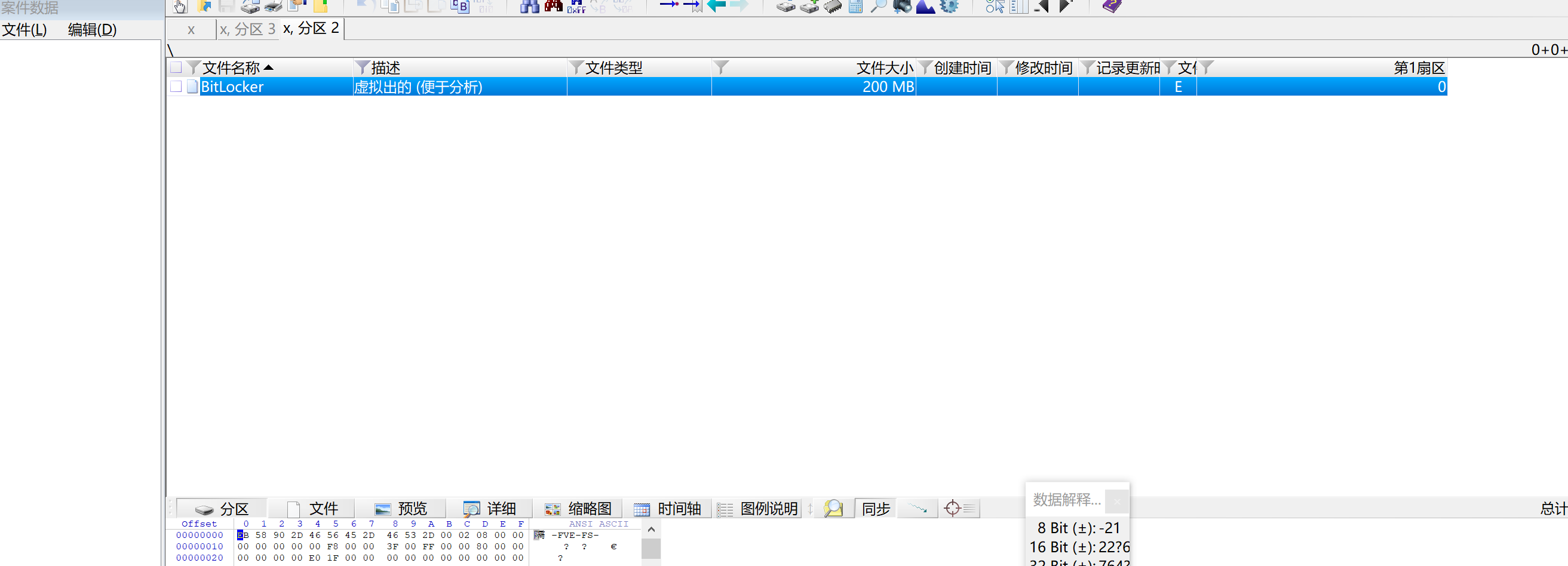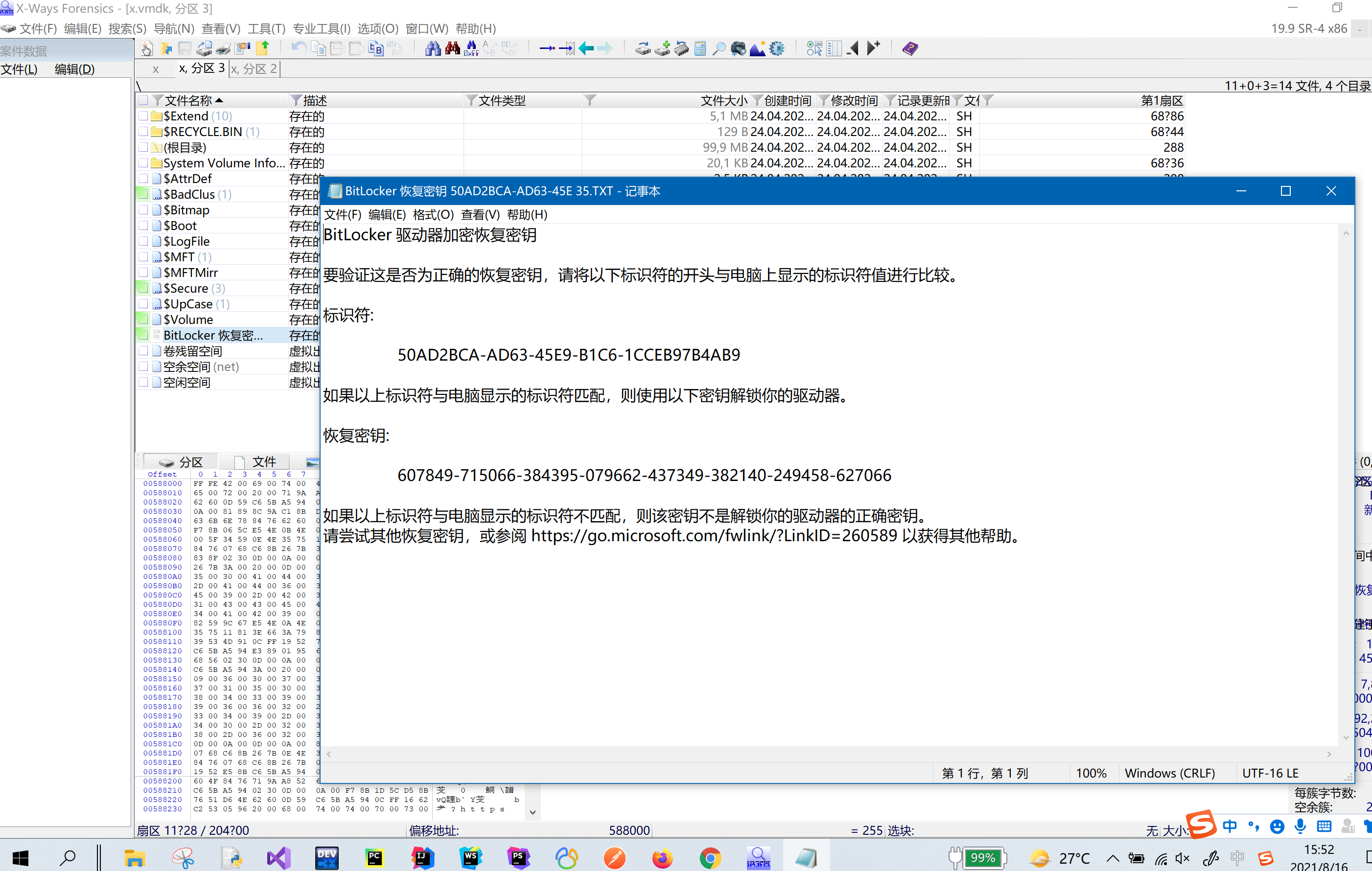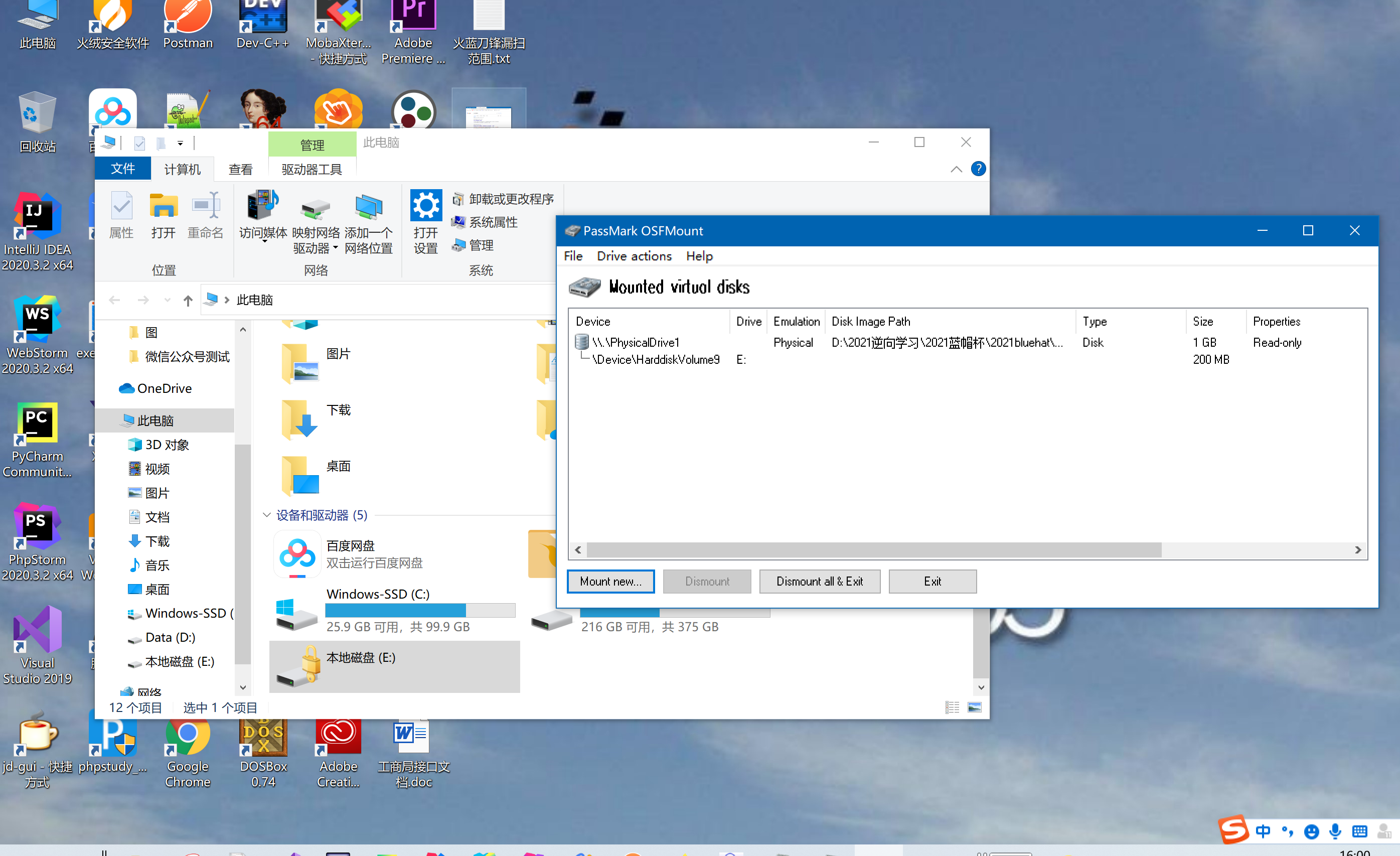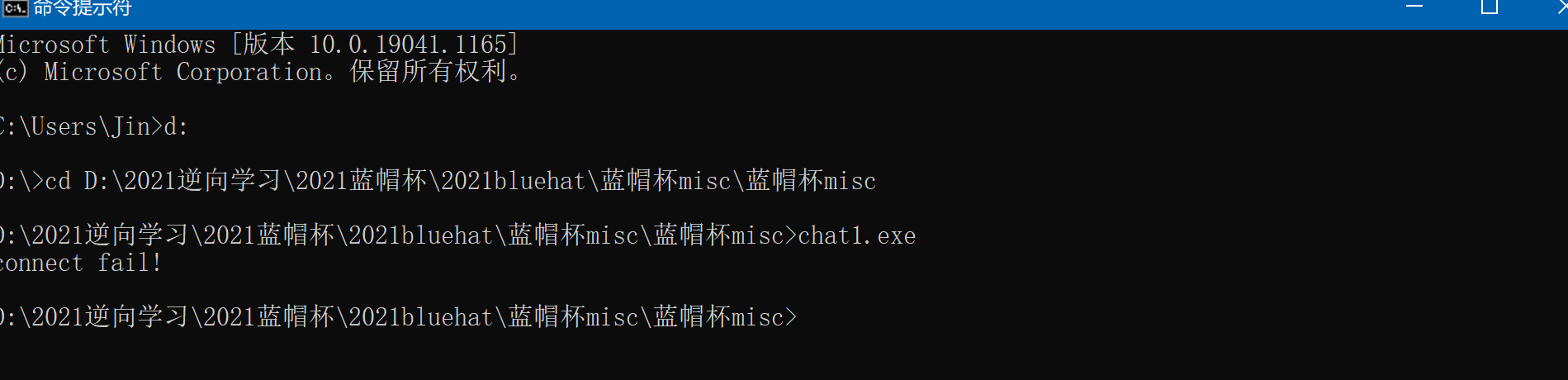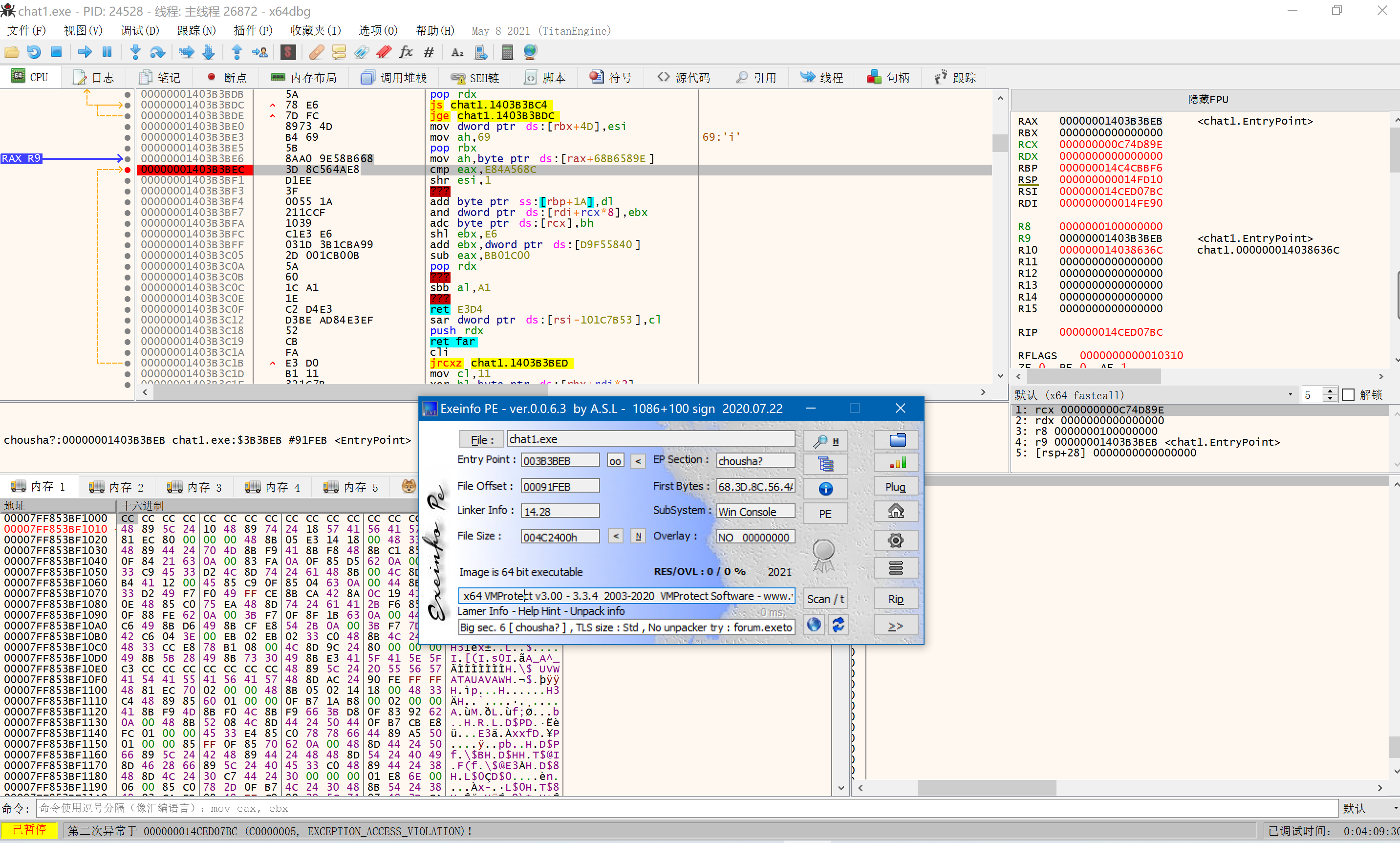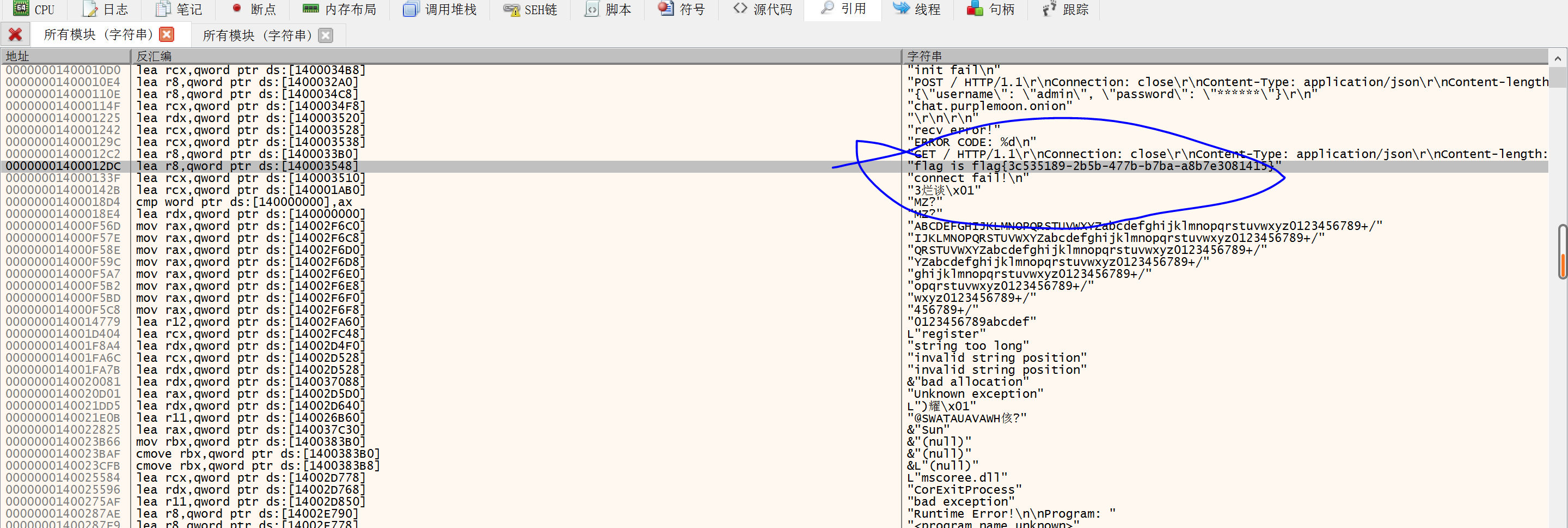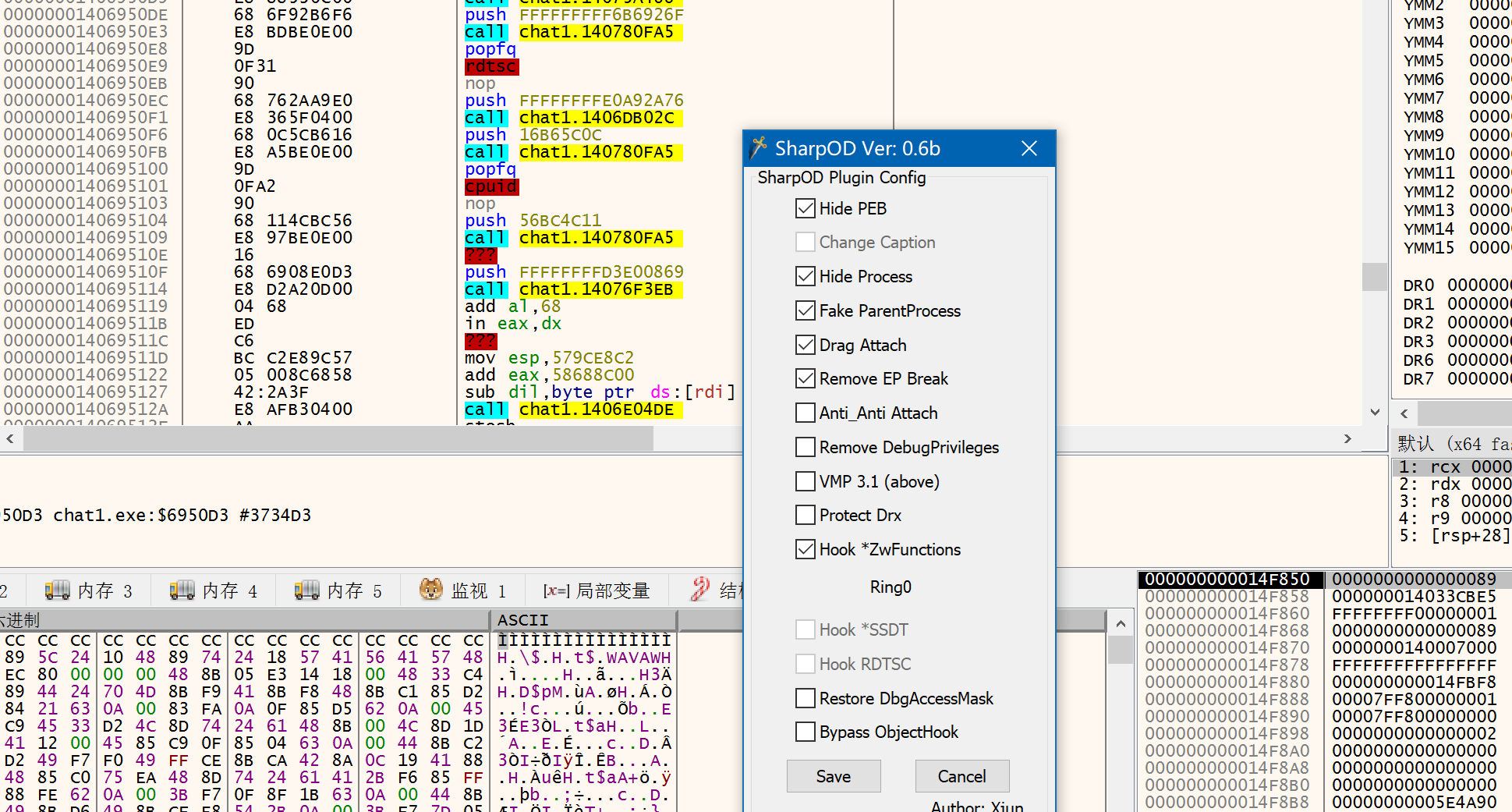##### 1、I_will_but_not_quite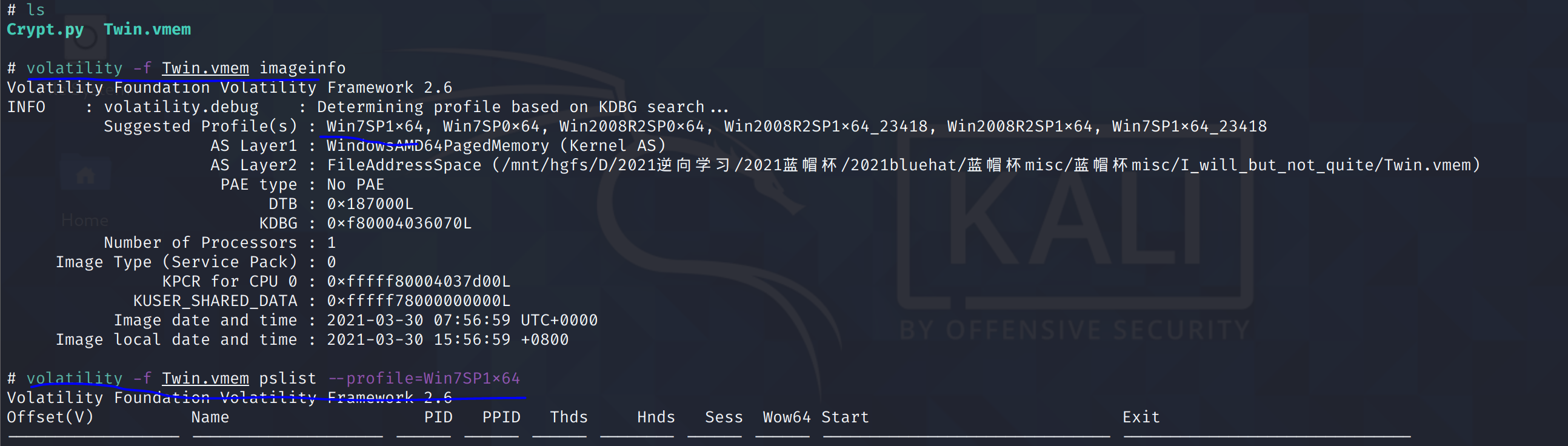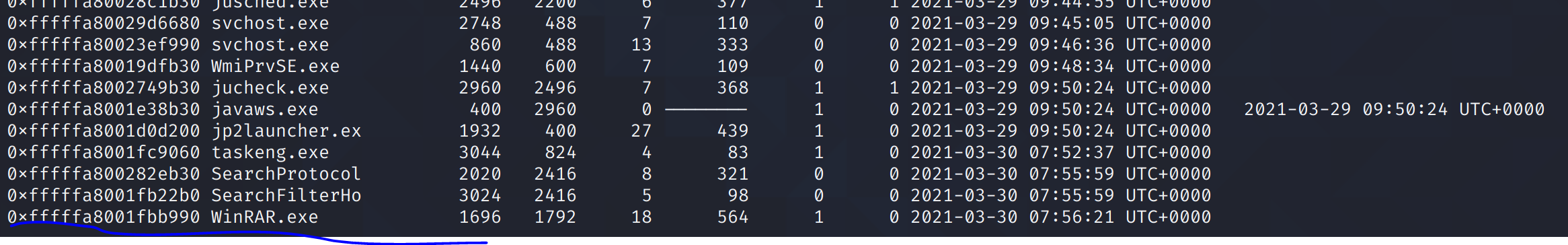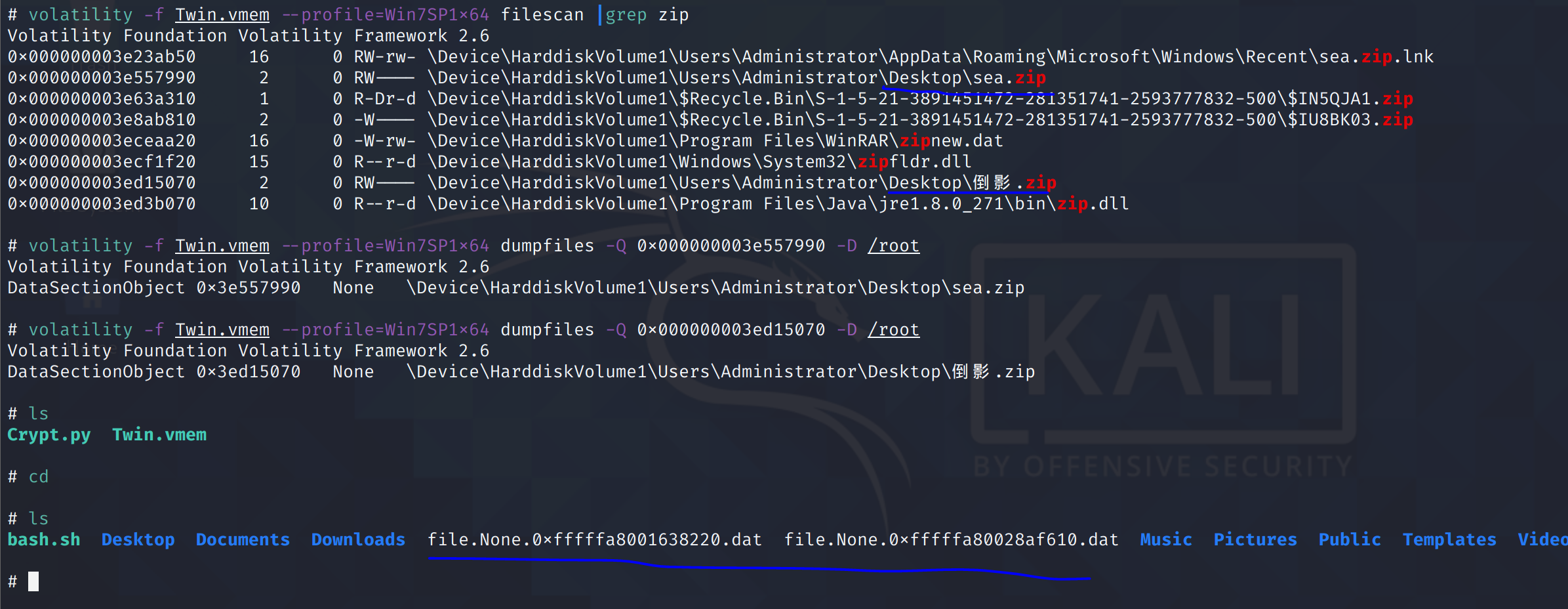``````./ etc/profile(个人原因)
volatility -f Twin.vmem(image name) imageinfo
volatility -f Twin.vmem pslist --profile=Win7SP1x64
volatility -f Twin.vmem --profile=Win7SP1x64 filescan |grep rar(zip)
volatility -f Twin.vmem --profile=Win7SP1x64 dumpfiles -Q 0x000000003e557990(pid) -D /root
``````

``````4266gj2zn17b2jo5b62k73g22xg6j658350r5771vd40h4bd2ns33q30651y57s6752su3q05881hs3h53nb3603co2mv40l58n3da3f61i5
``````

https://www.calcresult.com/misc/cyphers/twin-hex.html

``````Vnw3HC07BDgbBWNRGTx2fSckf399V1Z9CxIvHVd6fHsaEnR8fX40NyQ7JhM8CWV5fgMNN24=
``````
``````#!/user/bin/python2
import random
def r(s, num):
l=""
for i in s:
if(ord(i) in range(97,97+26)):
l+=chr((ord(i)-97+num)%26+97)
else:
l+=i
return l

def x(a, b):
return chr(ord(a)^ord(b))

def encrypt(c):
secret = c
n=random.randint(1,1000)
for i in range(n):
secret = r(secret, random.randint(1,26))
secret = secret.encode('base64')

l = ""
for i in range(len(secret)):
l += x(secret[i], secret[(i+1)%len(secret)])
return l.encode('base64')

flag = "#################"
print "secret =", encrypt(flag)

#secret = The key you got

``````

``````for i in range(86,128):
j = 1
tmp = [""]*len(dec)
tmp[-1] = chr(i)#爆破恢复最后一位，即可恢复所有
while j != len(dec):
tmp[-j-1] = chr(ord(dec[-j])^ord(tmp[-j])) #反着进行异或
j += 1
s = tmp[-1] #因为最后一位是最后一位和第一位异或，所以刚开始异或的其实是最后一位
for i in range(len(tmp)-1):
s += tmp[i]#这里即是将第2位至最后一位拼接起来加在第一位后面
``````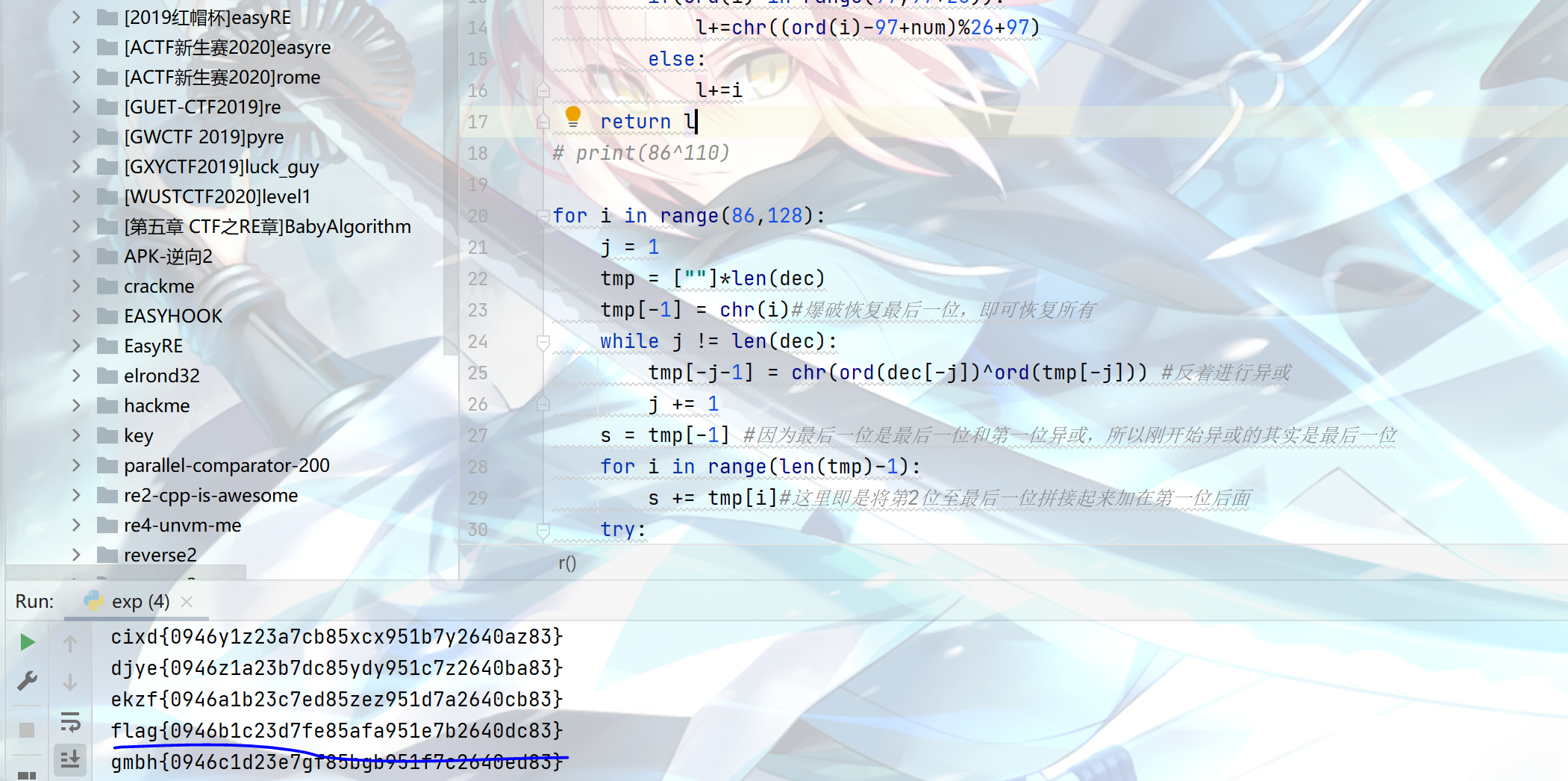##### 2、冬奥会_is_coming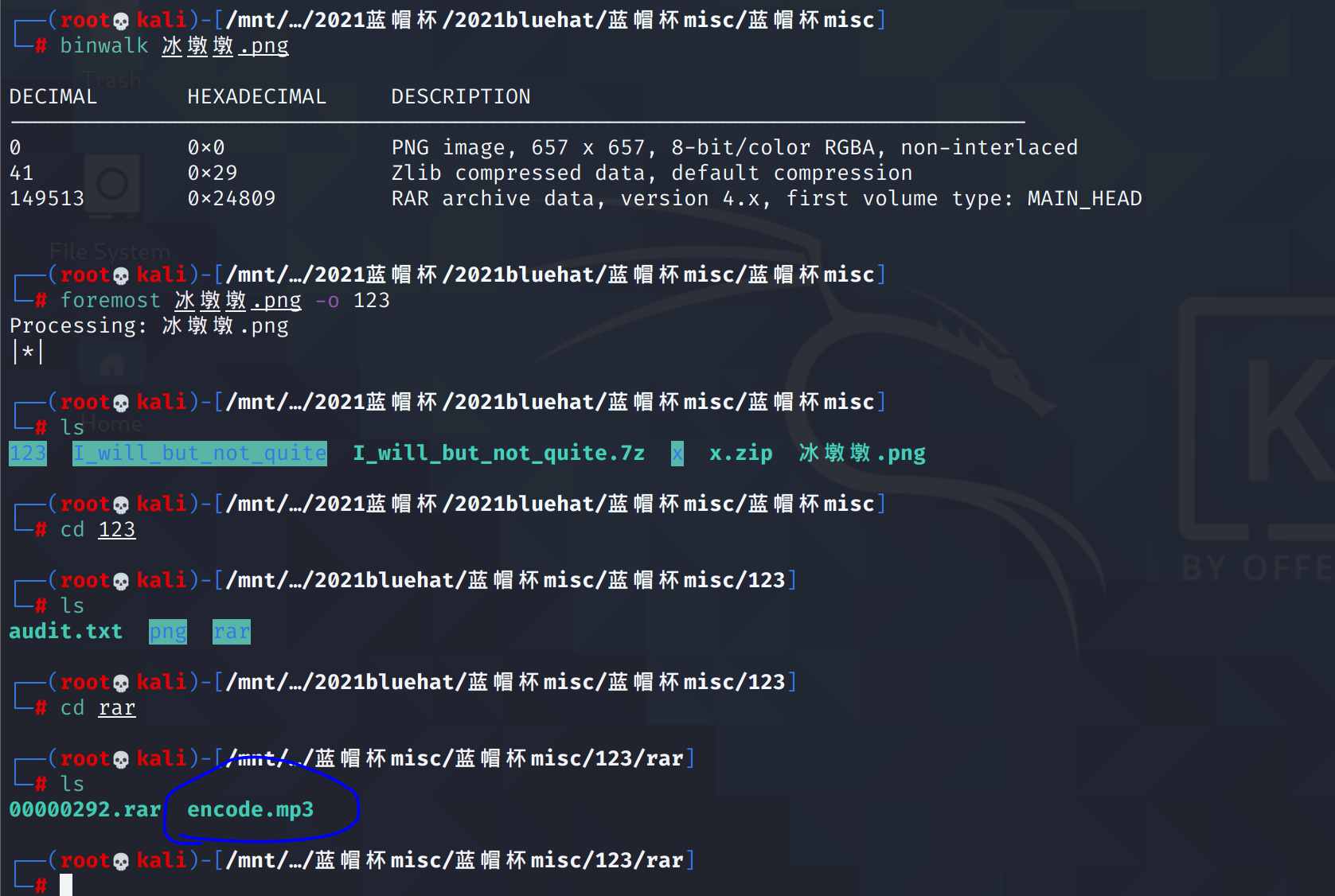rar压缩包里注释提示密码是8位数字，这里用mp3stego去解，因为题目不叫冬奥会 coming啥的么，所以猜测密码是冬奥会开幕日20220204,还不错，猜对了。然后就来了一串阴间的,这里做不下去了，看wp去了

``````Decode.exe -X -P 20220204 encode.mp3
``````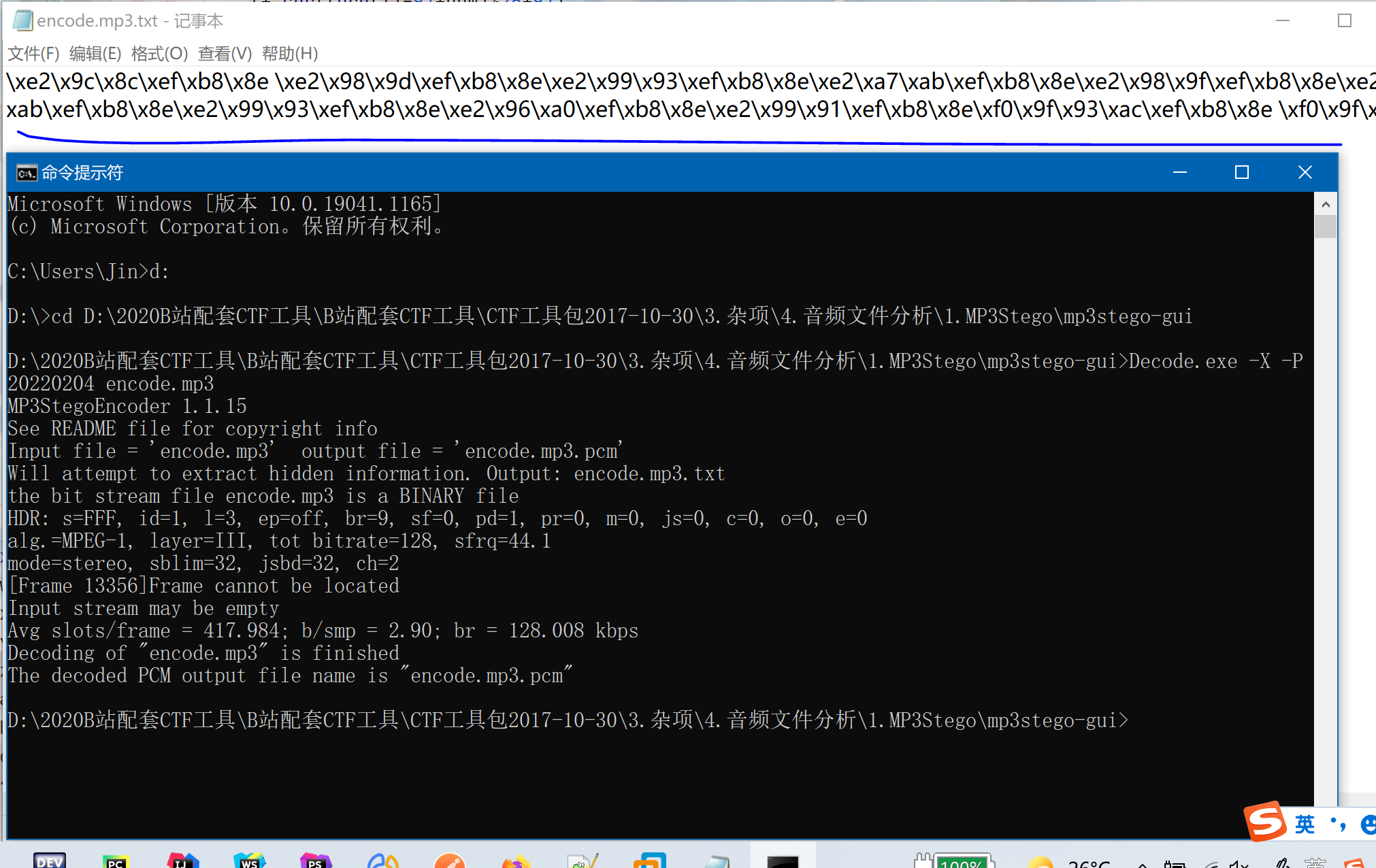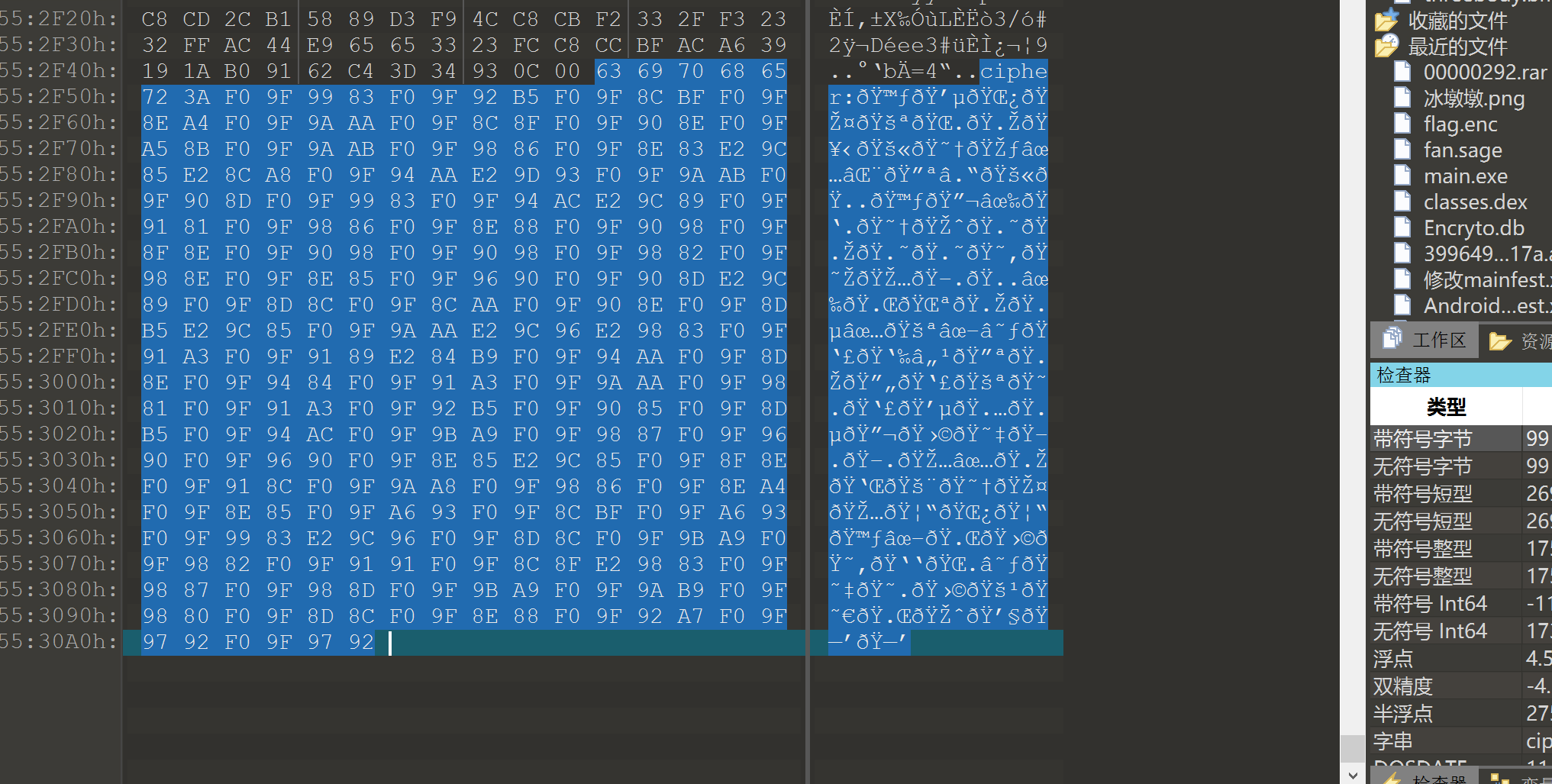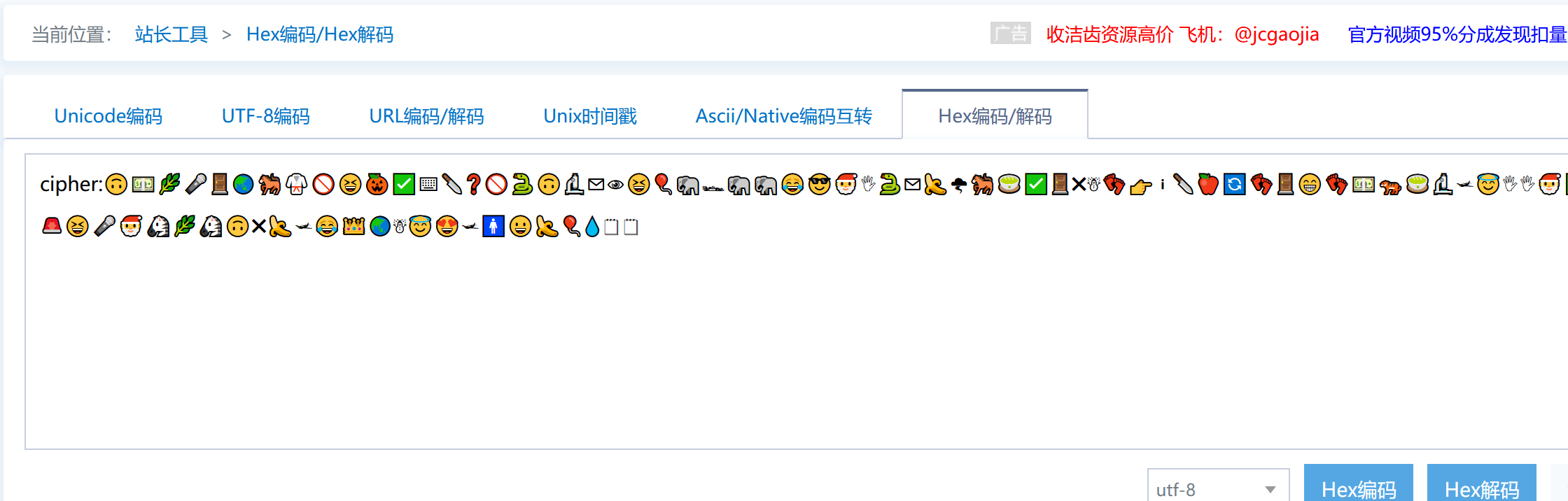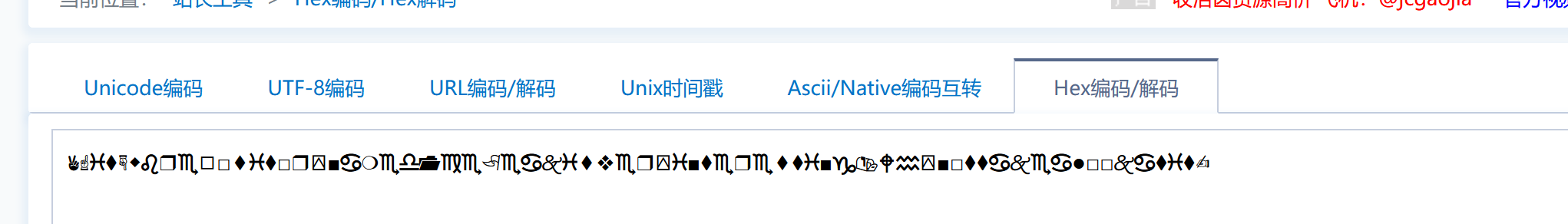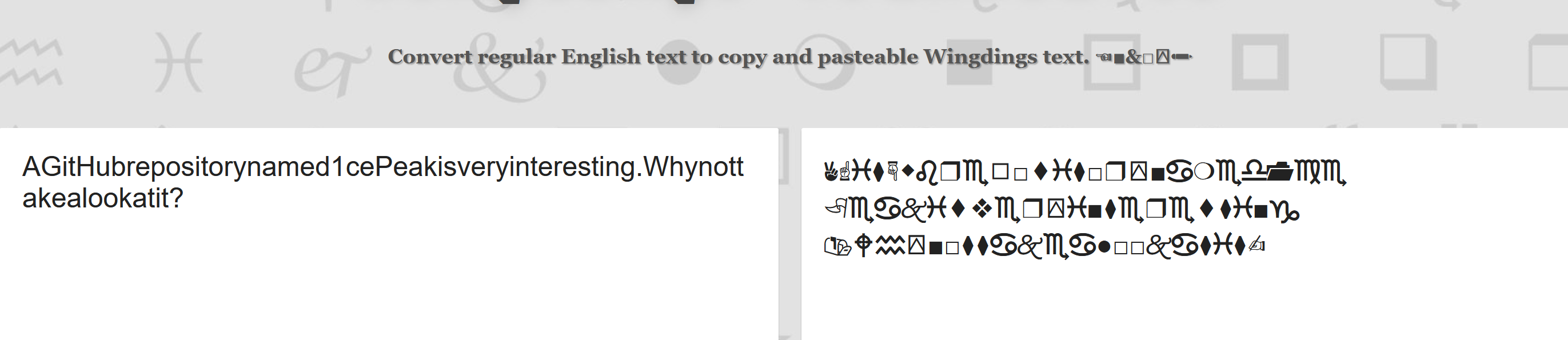https://aghorler.github.io/emoji-aes/

``````🙃💵🌿🎤🚪🌏🐎🥋🚫😆🎃✅⌨🔪❓🚫🐍🙃🔬✉👁😆🎈🐘🏎🐘🐘😂😎🎅🖐🐍✉🍌🌪🐎🍵✅🚪✖☃👣👉ℹ🔪🍎🔄👣🚪😁👣💵🐅🍵🔬🛩😇🖐🖐🎅✅🏎👌🚨😆🎤🎅🦓🌿🦓🙃✖🍌🛩😂👑🌏☃😇😍🛩🚹😀🍌🎈💧🗒🗒

flag{e32f619b-dbcd-49bd-9126-5d841aa01767}  服了
``````
##### 3、嫌疑人x的硬盘整理